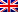# Searching in a tree (data structure)

To search in a graph, we first build a tree covering the graph.

Contenus

BFS browse by increasing depth to the root.

```BFS(Graph G, Node s): {f = CreateQueue (); f.stack (s); mark (s);
while no f.empty () s = f.pop (); print (s);
for each children t of s in G
yew t unmarked DO f.stack (t); mark (t);
end if
end for
end while
}```

## In-depth search: pre-order

In-depth algorithms are recursive. In the prefix path, we always browse the left subtree before processing the right subtree.

1. Check if the current node is empty or null.
2. Display the data part of the root (or current node).
3. Traverse the left subtree by recursively calling the pre-order function.
4. Traverse the right subtree by recursively calling the pre-order function.

## In-depth search: in-order

The LNR browse as far left as possible, and display the branches from left to right. This algorithm display the diagonals from bottom to top.

1. Check if the current node is empty or null.
2. Traverse the left subtree by recursively calling the in-order function.
3. Display the data part of the root (or current node).
4. Traverse the right subtree by recursively calling the in-order function.

## In-depth search: out-order

This is a diagonal browsing from top to bottom.

1. Check if the current node is empty or null.
2. Traverse the left subtree by recursively calling the post-order function.
3. Traverse the right subtree by recursively calling the post-order function.
4. Display the data part of the root (or current node).FRFRENRUES